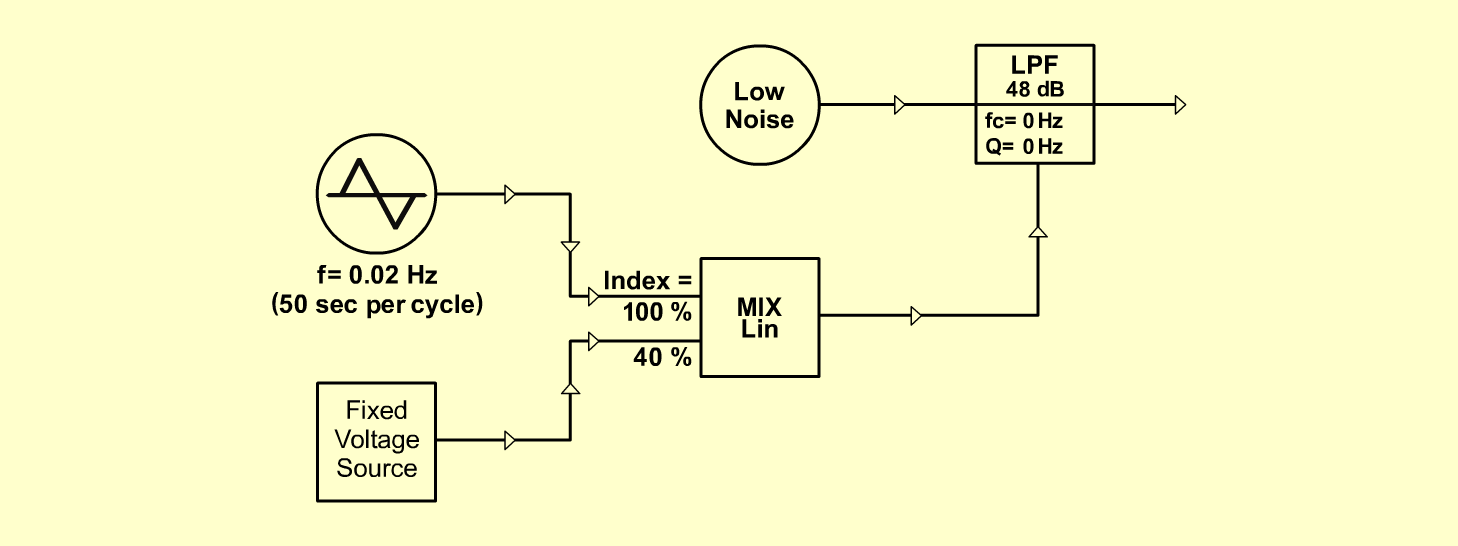Space ShuttleFXD o e p f e r A - 100 Connections: Settings: A-118 (Colored) <=> A-108 (Audio In) A-146 (Triangle) <=> A-138lin (Input 1) A-176 (CV 1) <=> A-138lin (Input 2) A-138lin (Output) <=> A-108 (CV 2) A-108 (48dB LP) <=> Amplifier A-118 (Blue = 10, Red = 10, Rate = 0, Level = 0) A-146 (Freq = 0, Wave Form = 5, Range = M) A-176 (CV 1 = 10, Fine = 10) A-138lin (In 1 = 10, In 2 = 4, In 3 = , In 4 = , Out = 10) A-108 (Freq = 0, CV 2 = 10, Emph = 0, Audio Level = 10) Josef MuellerSound sample Space Shuttle
br>